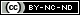## Thursday, March 3, 2016

### Chemistry for Preppers: Molecular Weights and Equations

Nature loves balance. When you start mixing chemicals together to form new compounds, you will need to make sure that you are using the right amounts to avoid wasting your supplies of reagents (reacting agents, aka “the chemicals you're using”). In order to do this, you'll need to know what you have, what you want to form, and some dreaded math.

The act of balancing out a chemical equation is known as stoichiometry, which is simply making sure that matter is not assumed to be created or lost, a clear violation of the laws of thermodynamics. When writing chemical equations, the number of atoms of each element must match on each side of the arrow in an equation. There is no right way to figure out how to balance and equation; trial and error work quite well.

Stoichiometry
Here's a simple equation: two nasty poisons, hydrochloric acid and sodium hydroxide. One is a strong acid and the other a strong base, so we know that when we mix them we will get a salt and some heat out of the reaction.
HCl + NaOH → H2O + NaCl
Yes, by mixing two things that will burn you, we have made salt water that you could bathe in -- just so long as you measured everything out correctly. Any leftover acid or base will make it dangerous, just like measuring out the wrong amounts of salt or flour can make a recipe inedible.

Another method would be to use pure Sodium metal (Na), which spontaneously bursts into flame when it contacts water, and Chlorine gas (Cl2) which is a poison gas used in WW1. This reaction releases a lot more energy as the ionic bonds are formed.

We know we have Na and Cl (stable as Cl2), and we know that NaCl will be the product. How do we balance the equation?
Na + Cl2 → NaCl
That doesn't work because there is a leftover Cl atom. We need more Na, so let's try using two of them.
2(Na) + Cl2 → 2(NaCl)
That works out, making everything balanced.

Notice the use of parentheses to show the presence of two atoms that are not bound together, similar to the mathematical use of parentheses to show multiplication, like 3(x2 + 2x + 5)... I warned you that there would be math.

Moles
So how do you measure out molecules? In chemistry, we use a standard unit of measurement, the mole (symbol is mol). One mole of anything (atoms, ions, tennis balls, cats, etc.) is roughly 6.023 x 1023. This may sound like an arbitrary number, but it was calculated and verified through experiments about 200 years ago. Known as Avogadro's number (or constant), it is named after Amedeo Avogadro who was an Italian lawyer, physicist, mathematician, and general experimenter in the early-mid 1800's.

Remember the periodic table and the atomic weight of each element? That number is how many grams one mole (g/mol) of that element will weigh. Add up the atomic weights of all of the constituents of a molecule and you get its molecular weight.

Example 1: NaCl
• The atomic weight of Na is 23.00 g/mol
• The atomic weight of Cl is 35.45 g/mol
• Therefore the molecular weight of NaCl is 58.45 g/mol

If you have a more complex molecule like sucrose (common sugar), you'll need to include the multiples of atoms present in the molecule (C12H22O11) so:

Example 2: C12H22O11
• 12 x C = 12 x 12.00 g/mol = 144.00 g/mol
• 22 x H = 22 x 1.00 g/mol = 22.00 g/mol
• 11 x O = 11 x 16.00 g/mol = 176.00 g/mol
• So the molecular weight = 342.00 g/mol

Now when you run across a chemical equation, you can consult your periodic table and figure out how much of each chemical you're going to need to weigh out to get the amount of product you need, or how much of something is going to be produced from a given amount of a reagent.

This should be the last of the basic chemistry posts, with an occasional not-Thursday post about chemistry-related things. Next week we'll get into electrochemistry and how batteries (cells, actually) work. Electroplating of metals may be a separate post if there is any interest in the topic.

I also have drafts for several physics (light, sound, momentum, etc.) posts floating around in my to-do list; we'll have to see if they can be beaten into something worth posting.

If there is anything in particular that you would like me to cover, please let me know and I'll give it some thought. Be warned that I won't be posting much about DIY explosives or drug manufacturing. Besides being blatantly illegal, I don't need the liability from experiments beyond my control!